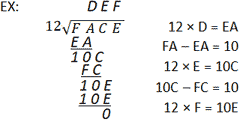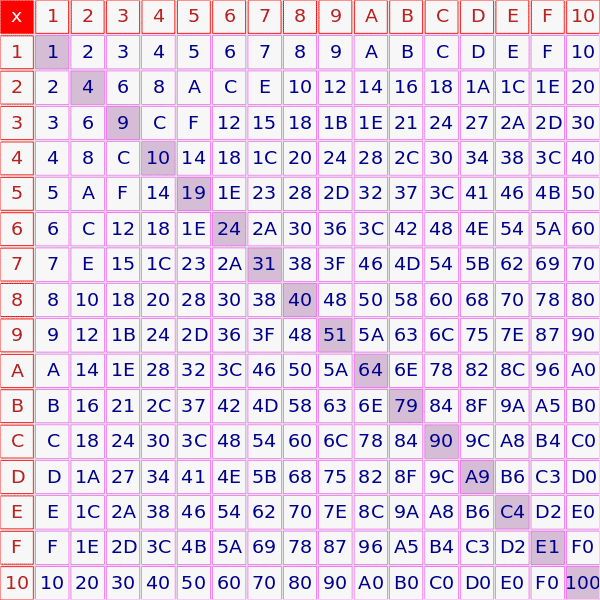# Hex Calculator

## Result

Hex value:
7ffee0fca840 + 14 = 7FFEE0FCA854

Decimal value:
140732673075264 + 20 = 140732673075284

 + - × ÷ = ?## Convert Hexadecimal Value to Decimal Value

 Hexadecimal Value: = ?## Convert Decimal Value to Hexadecimal Value

 Decimal Value: = ?RelatedBinary Calculator | IP Subnet Calculator

The hexadecimal number system (hex) functions virtually identically to the decimal and binary systems. Instead of using a base of 10 or 2 respectively, it uses a base of 16. Hex uses 16 digits including 0-9, just as the decimal system does, but also uses the letters A, B, C, D, E, and F (equivalent to a, b, c, d, e, f) to represent the numbers 10-15. Every hex digit represents 4 binary digits, called nibbles, which makes representing large binary numbers simpler. For example, the binary value of 1010101010 can be represented as 2AA in hex. This helps computers to compress large binary values in a manner that can be easily converted between the two systems.

Below are some typical conversions between hex, binary, and decimal values:

Hex/Decimal Conversion

 Hex Binary Decimal 0 0 0 1 1 1 2 10 2 3 11 3 4 100 4 5 101 5 6 110 6 7 111 7 8 1000 8 9 1001 9 A 1010 10 B 1011 11 C 1100 12 D 1101 13 E 1110 14 F 1111 15 14 10100 20 3F 111111 63

Converting between decimal and hex involves understanding the place values of the different number systems. A more in depth discussion is available on the binary calculator page. Note that converting between decimal and hex is quite similar to converting between decimal and binary. The ability to perform the conversion of either should make the other relatively simple. As previously mentioned, hex functions using the base of 16. This means that for the value 2AA, each place value represents a power of 16. Starting from the right, the first "A" represents the "ones" place, or 160. The second "A" from the right represents 161, and the 2 represents 162. Remember that "A" in hex is equivalent to 10 in decimal. Knowing this information, it is then possible to convert from hex to decimal, as shown below:

 EX: 2AA = (2 × 162) + (A × 161) + (A × 160) = (2 × 256) + (10 × 16) + (10 × 1) = 512 + 160 + 10 = 682

Converting from decimal to hex is slightly more involved, but uses the same concepts. Refer to the steps and example below. It is important to work through the example provided in conjunction with the listed steps in order to understand the process:

1. Find the largest power of 16 that is less than or equal to the number to be converted, which will be referred to as X.
2. Determine how many times the power of 16 found in Step 1 goes into X, and take note of that number.
3. Multiply the number found in Step 2 by the power of 16 and subtract this value from X. This new value will be referred to as Y.
• Note that the number found in Step 2 will be the value written in the place value for the power of 16 that was found. If for example the largest power of 16 was found to be 164, and the number in Step 2 found to be 3, the hex value would have the number 3 in its 164 place value: 3qrst, where qrst represents the 160 through 3 place values.
4. Repeat Steps 1-3 using Y as the new starting value. Continue the process until 16 is larger than the remaining value, and assign the remainder to the 160 place value.
5. Assign each of the values found in each iteration of Step 2 to its respective place value to determine the hex value.
 EX: Convert decimal 1500 to hex (1) Largest power = 162 = 256 (2) 256 × 5 = 1280, so (5 × 162) (3) 1500 - 1280 = 220 (4) 16 × 13 = 208, so (13 × 161) (5) 220 - 208 = 12 (6) 16 is larger than 12, so 12 is the value in the 160 place value (7) 1500 = (5 × 162) + (13 × 161) + (12 × 160) (8) Remember that 10-15 have letter numerals In hex: 13 = D, and 12 = C (9) Therefore the hex value of 1500 is: 5DC

Converting from hex to decimal utilizes the same principles, but is arguably simpler. Multiply each digit in the hex value by its corresponding place value, and find the sum of each result. The process is the same regardless of whether the hex value contains letter numerals or not.

 EX: Convert hex 1024 to decimal (1) (1 × 163) + (0 × 162) + (2 × 161) + (4 × 160) (2) 4096 + 0 + 32 + 4 = 4132

Hex addition follows the same rules as decimal addition with the only difference being the added numerals A, B, C, D, E, and F. It may be convenient to have the decimal equivalent values of A through F handy when performing hex operations if the values have not yet been committed to memory. Below is an example of hex addition. Work through the example, and refer to the text below it for further details.

EX:
 18 1A B + B 7 8 = 1 4 2 3

Hex addition involves calculating basic decimal addition while converting between hex and decimal when values larger than 9 (the numerals A through F) are present. In the example above, B + 8 in decimal is 11 + 8 = 19. 19decimal is 13hex, since there is 1 set of 16, with 3 left over. Just like in decimal addition, the 1 carries over to the next column. Hence, the next column works out to be 1 + A (10) + 7 = 18decimal, or 12hex. Carry over the 1 to the final column resulting in 1 + 8 + B (11) = 20decimal, or 14hex. This yields the result of 1423hex.

### Hex Subtraction

Hex subtraction can be computed much the same way as hex addition; by performing the operation while converting between hex and decimal values. The most significant difference between hex and decimal subtraction involves borrowing. When borrowing in hex, the "1" that is borrowed represents 16decimal rather than 10decimal. This is because the column that is being borrowed from is 16 times larger than the borrowing column (the same reason that the borrowed 1 in decimal represents 10). As long as this is noted, and conversions of the letter numerals A-F are done carefully, hex subtraction is not any more difficult than decimal subtraction. Work through the example, and refer to the text below it for further details.

EX:
 5 D 1C – 3 A F = 2 2 D

In the first column on the right of the above example, C, or 12decimal, is smaller than F, or 15decimal. As such, it is necessary to borrow from the next column. This reduces the D, to C, and lends 1, or 16decimal to the first column. 16decimal + 12decimal - 15decimal - 13decimal, or D in the first column. The following columns require no borrowing, making the calculations simple. Since 1 was borrowed, C - A = 12decimal - 10decimal = 2, and 5 - 3 = 2 yielding the final result of 22D. In the case where the number being subtracted is larger than the number being subtracted from, simply change the positions of the numbers, calculate the subtraction, and add a negative sign to the result. If the above example were instead 3AF - 5DC, it would then be written as is, except that the solution would be -22D.

### Hex Multiplication

Hex multiplication can be tricky because the conversions between hex and decimal when performing the operations require more effort since the numerals tend to be larger. Having a hexadecimal multiplication table can be helpful (one is provided below).Otherwise manual conversion between decimal and hex will be necessary for each step. Below is an example of hex multiplication. To the right of the example, each of the multiplication and addition steps are shown. Note that all of the numerals used are hex. Refer to the addition section if necessary.

EX:
 F A 3 × A = 1E; 1 carried to F × C 3 3 × F = 2D, + 1 = 2E 2 E E C × A = 78; 7 carried to F + B B 8 0 C × F = B4, + 7 = BB = B E 6 E

### Hex Division

Long division in hex is identical to long division in decimal, except that the multiplication and subtraction occur in hex. It is also possible to convert to decimal and perform long division in decimal, then convert back once complete. For illustrative purposes, the division example will be calculated entirely in hex. As with multiplication, having a hexadecimal multiplication table (one is provided below) would be convenient while conducting hex division. Below is an example. Note that all numerals in the example are hex. Although no borrowing occurs in the example below, remember that borrowing in hex results in 16decimal being borrowed, rather than 10decimal. Refer to the hex subtraction section for further details.